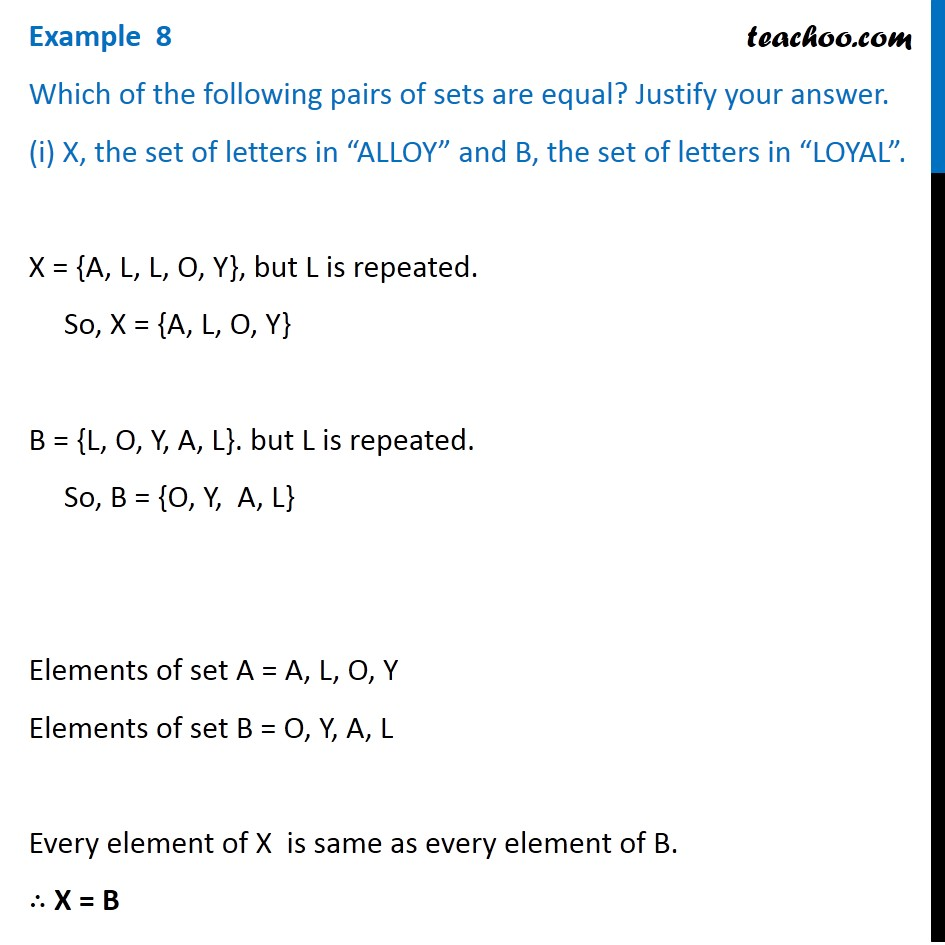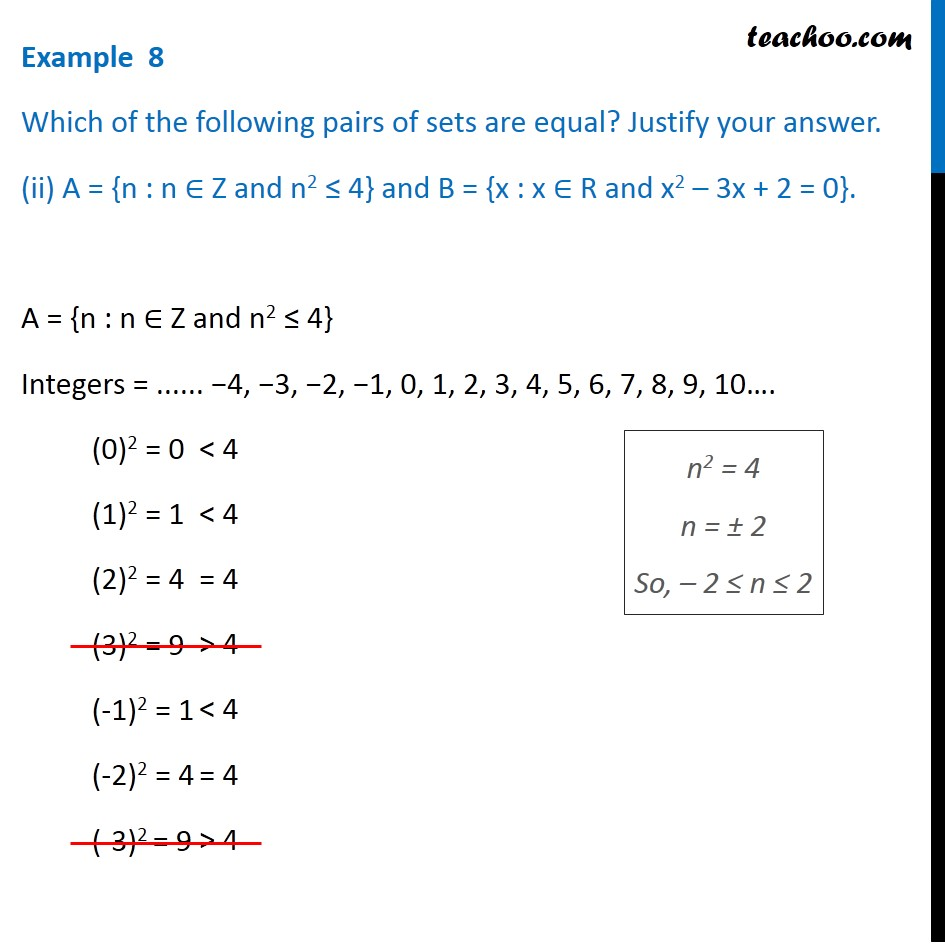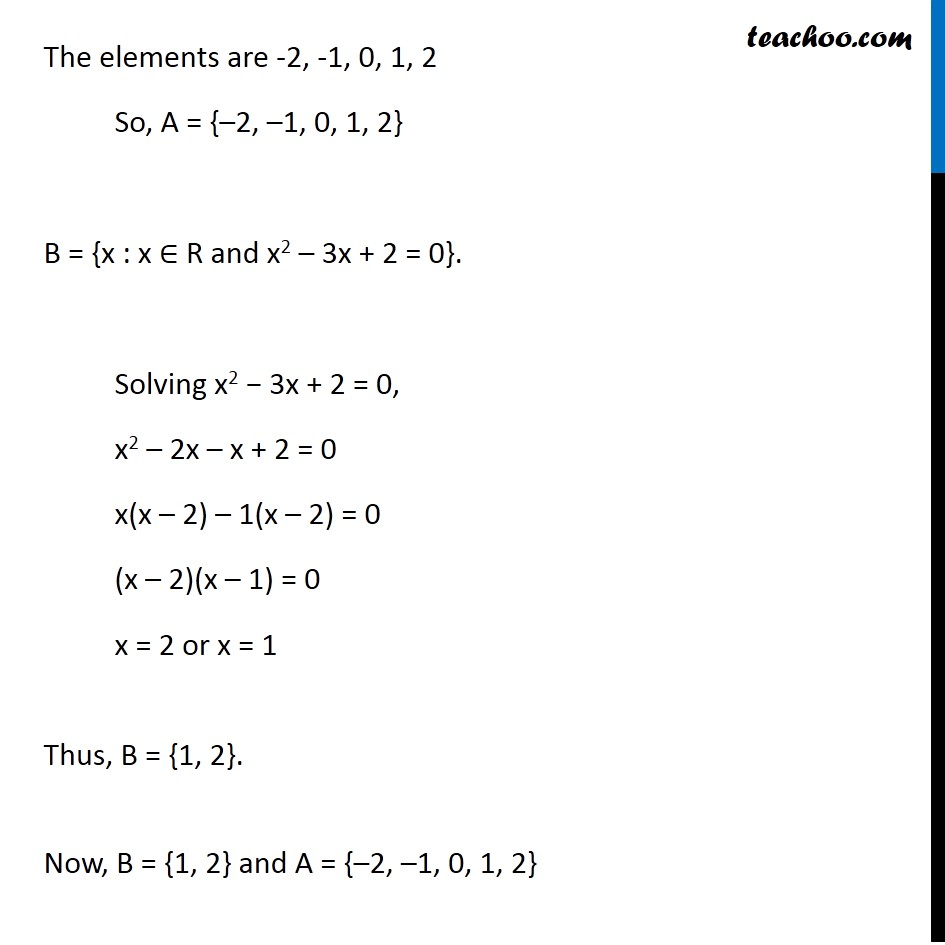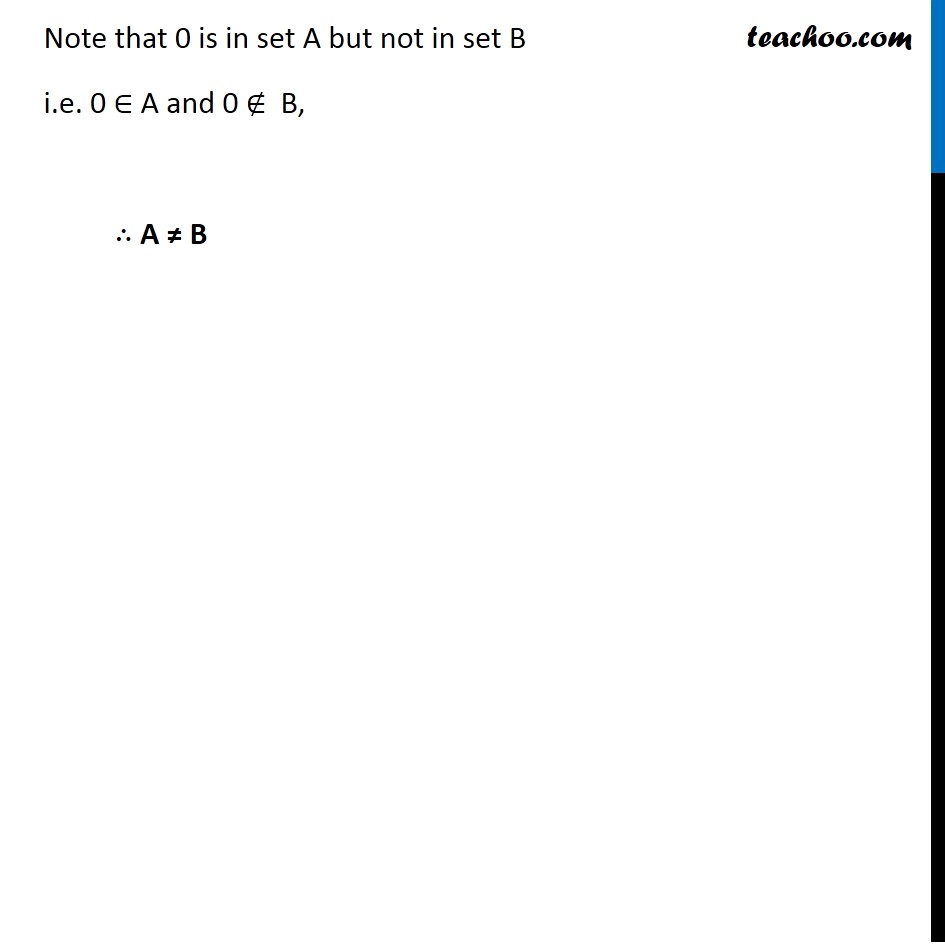1. Chapter 1 Class 11 Sets
2. Serial order wise
3. Examples

Transcript

Example 8, Which of the following pairs of sets are equal? Justify your answer. (i) X, the set of letters in ALLOY and B, the set of letters in LOYAL . X = {A, L, L, O, Y}, but L is repeated. So, X = {A,L,O,Y} B = {L, O, Y, A, L}. but L is repeated. So, B = {O,Y, A,L} Elements of set A = A, L, O, Y Elements of set B = O, Y, A, L Every element of X is same as every element of B. X = B

Examples

Chapter 1 Class 11 Sets
Serial order wise